# Triangle TypesPage 1

#### WATCH ALL SLIDES

Slide 1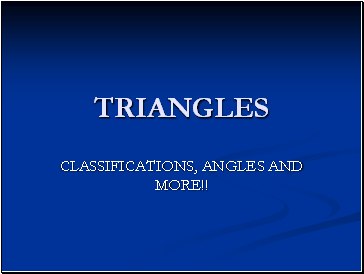TRIANGLES

CLASSIFICATIONS, ANGLES AND MORE!!

Slide 2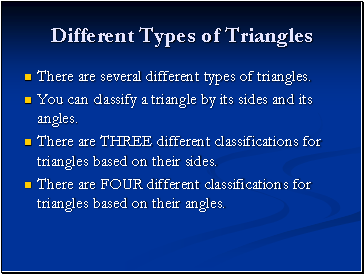## Different Types of Triangles

There are several different types of triangles.

You can classify a triangle by its sides and its angles.

There are THREE different classifications for triangles based on their sides.

There are FOUR different classifications for triangles based on their angles.

Slide 3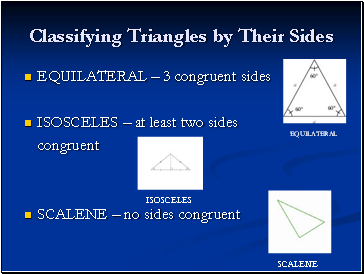## Classifying Triangles by Their Sides

EQUILATERAL – 3 congruent sides

ISOSCELES – at least two sides

congruent

SCALENE – no sides congruent

EQUILATERAL

ISOSCELES

SCALENE

Slide 4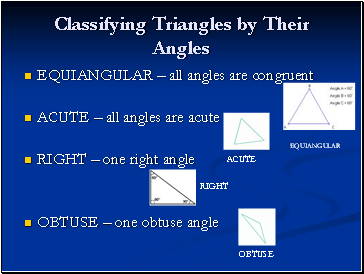## Classifying Triangles by Their Angles

EQUIANGULAR – all angles are congruent

ACUTE – all angles are acute

RIGHT – one right angle

OBTUSE – one obtuse angle

EQUIANGULAR

ACUTE

RIGHT

OBTUSE

Slide 5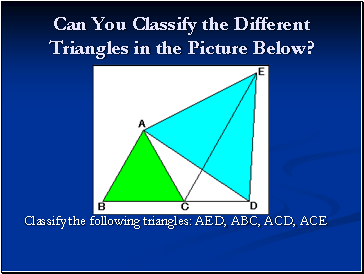## Can You Classify the Different Triangles in the Picture Below?

Classify the following triangles: AED, ABC, ACD, ACE

Slide 6## The Classifications…

Triangle AED = Equilateral, Equiangular

Triangle ABC = Equilateral, Equiangular

Triangle ACD = Isoceles, Obtuse

Triangle ACE = Scalene, Right

So how did you do?